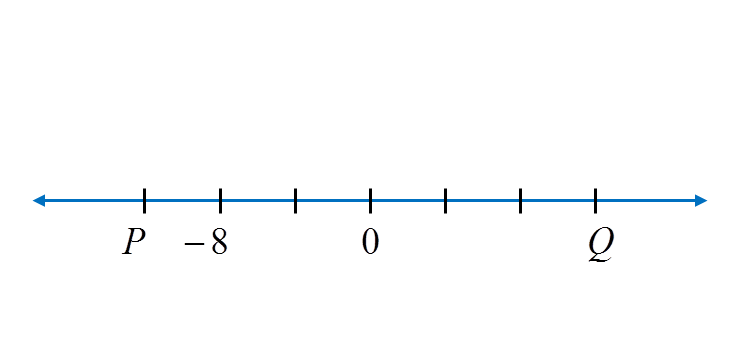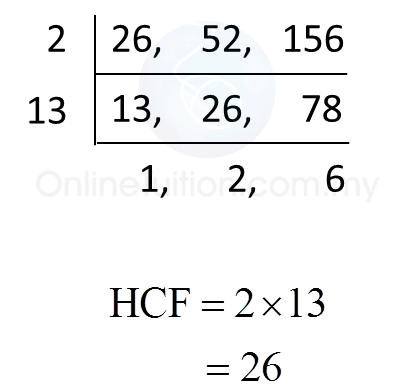# 2.2.2 Number Patterns and Sequences, PT3 Practice

               
Question 6:
 How many prime numbers are there between 10 and 40?
 

 Solution:
 
Prime numbers between 10 and 40
 
= 11, 13, 17, 19, 23, 29, 31, 37
There are 8 prime numbers between 10 and 40.
 
 
 
               
Question 7:
 
Diagram below shows a sequence of prime numbers.
               Find the value of x + y.
 
 Solution:
 
Value of x + y
 
= 19 + 23
 = 42

 
               
Question 8:
 
The following data shows a sequence of prime numbers in ascending order.
               Find the value of x, y and of z.
 
 Solution:
 
x = 11, y = 31, z = 37
 
 
 
       
Question 9:
 
The following data shows a sequence of prime numbers in ascending order.
           Find the value of p, q and of r.
 
 Solution:
 
p = 59, q = 71, r = 79
 
 

 
 
       
Question 10:
 
Diagram below shows several number cards.
            List three prime numbers from the diagram.
 
 Solution:
 
Prime number card from the diagram = 5, 29 and 97.
 
 

 
 

# 2.2.1 Number Patterns and Sequences, PT3 Practice

Question 1:
Diagram below is part of a number line.What is the value of P and of Q?

Solution:
Each gradation on the number line represents 4 units. Thus P = –12 and Q = 12.

Question 2:
Diagram below shows a sequence of numbers. K and M represent two numbers.
What are the values of K and M?

Solution:K
= 13 – 6 = 7
M = 1 – 6 = –5

Question 3:
Diagram below shows five integers.Find the sum of the largest integer and the smallest integer.

Solution:
The largest integer = 1
The smallest integer = –10
Sum of the largest integer and the smallest integer
= 1 + (–10)
= –9

# 2.2.4 Number Patterns and Sequences, PT3 Practice

 [adinserter block="3"]       
Question 16:
 State the first two common multiples of 3, 5 and 6.
 
 Solution:
 
Multiples of 3 = 3, 6, 9, 12, 15, 18, 21, 24, 27, 30, 33, 36, 39, 42, 45, 48, 51, 54, 57, 60, …
 Multiples of 5 = 5, 10, 15, 20, 25, 30, 35, 40, 45, 50, 55, 60, …
 Multiples of 6 = 6, 12, 18, 24, 30, 36, 42, 48, 54, 60, …
 
 The first two common multiples of 3, 5 and 6 are 30 and 60.
 
 
 
 [adinserter block="3"]       
Question 17:
 Find the lowest common multiple (LCM) of 4, 8 and 24.
 
 Solution:[adinserter block="3"]       
Question 18:
 Find the lowest common multiple (LCM) of 8, 12 and 15.
 

 Solution:[adinserter block="3"]   
Question 19:
 Find the highest common factor (HCF) of 26, 52 and 156.
 

 Solution:[adinserter block="3"]   
Question 20:
 Given that (m + 5) is the highest common factor of 81 and 108, find the value of m.
 

 Solution:# 2.2.3 Number Patterns and Sequences, PT3 Practice

[adinserter block="3"]
Question 11:
State all the prime factors of 156.

Solution:

[adinserter block="3"]
Question 12:
State all the prime factors of 182.

Solution: